Disclaimer: This is an example of a student written essay.

Any opinions, findings, conclusions or recommendations expressed in this material are those of the authors and do not necessarily reflect the views of UKEssays.com.

# Performance Analysis of One Dimension FDTD Code

 ✅ Paper Type: Free Essay ✅ Subject: Computer Science ✅ Wordcount: 2243 words ✅ Published: 7th Aug 2018

#### Performance analysis of One dimension FDTD code using Parallel Processing Technique

• P. GUNAPANDIAN, M.R.SUBASREE, B. MANIMEGALAI

### Abstract

The requirement of longer processing time and larger memory makes FDTD method impractical for many cases. Implementation of parallel processing in FDTD method is proposed in this paper. The implementation depends mainly on computer architecture and programming libraries under different operating systems. In this paper a several performance tests of a one dimension FDTD code is tested in different platforms. The results shows that Parallel processing shows a linear decrease in time and larger data handling which makes it as a right platform for complex structures.

Keywords: FDTD, parallel processing, computer architecture, operating system, serial processing.

## Introduction

Computational ability has advanced in the recent few decades. One of the most common methods to solve Maxwell’s equations on arbitrary configurations of materials and field sources is the Finite Differences on Time Domain (FDTD) . There exist a number of techniques for enhancing the performance of the conventional FDTD, in order to obtain accuracy the conformal FDTD method which simulates the curved perfect electrical conductor (PEC) [2,3]. Sub-gridding technique is used to increase the mesh density in the local area in which the field varies quickly [5,6]. The Multi-Resolution Time-Domain (MRTD) and Pseudo-Spectrum Time-Domain (PSTD) techniques are used to reduce the dispersion of the conventional yee grid [7,8]. The above techniques mentioned are used to improve the conventional FDTD technique for the purpose of reducing either memory requirements or simulation time. The parallel-processing FDTD accelerates the FDTD simulation by distributing the job to multiple processors, so that the available memory for large problems is virtually unlimited. At the same time, the simulation time is dramatically reduced compared to a single-processor implementation. On the computational point of view the parallel processing have an important advantage which makes the parallel executions strategies easier.

If you need assistance with writing your essay, our professional essay writing service is here to help!

Parallel processing in computers is based on dividing a computer code into a number of segments and distribution of the task among a number of computers/processors, which are then executed in parallel. This may be achieved on hardware-level, software level, or both. Hardware-level parallelization necessitates alternative processor designs. Software-level parallelization can be either on data level or function level, depending on the characteristics of the code .

In this paper one dimensional FDTD code is developed for parallel processing. The performance analysis of the code developed is compared with both serial and parallel. The code is simulated in different platforms and the results are obtained. The results shows the efficiency of the parallel processing in handling larger data and reduction of time compared to serial processing.

## FDTD METHOD

The FDTD method is one of the well known approaches to solve Maxwell’s partial differential equations, because of its high versatility. FDTD algorithm is based on temporal and three-dimensional spatial discretization and it transforms the time-dependent Maxwell’s curl equations into a set of finite-difference relations [10, 11].Boundary conditions are needed on the edge of the simulation domain, among the several possible choices perfect matched layer (PML) boundary can be more accurate and the PML boundary is used in the developed code.

### One Dimension FDTD

The one dimension FDTD starts with the formulation of the Maxwell’s equations,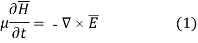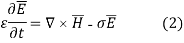Where E and H are electric and magnetic field respectively. The one dimension equation for Ex and Hy are given, which denotes the Electric field with respect to x direction and magnetic field with respect to y direction. The update equations are given in (3) and (4).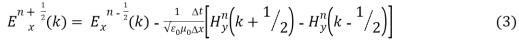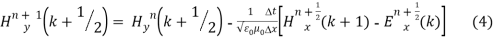The FDTD update equations are used to develop the Matlab code and the code is excited with the Gaussian pulse and the results are obtained.

## PARALLEL FDTD

According to the principle of FDTD algorithm, the electromagnetic field value at certain position can be decided by the value of last time step at this position and electromagnetic field value of this time step at nearby position. The electromagnetic field value has no direct relation to the values at position far from this point. So, the whole computational space can be divided into some sections that can be computed in some nodes of parallel computing system. The exchange of field values between nodes can be executed only at interface between sections. According to the basic concept, the computing between parallel nodes can be executed to simulate the serial computing in a single PC or workstation. This is the key point of our parallel FDTD algorithm. Fig.1 shows the methodology of serial and the parallel approach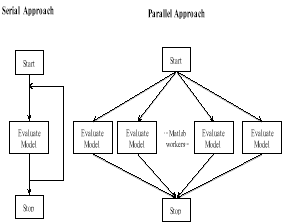Figure 1. Serial and parallel approach

## PARALLEL PROCESSING TECHNIQUE

The main idea of parallel processing starts with the updating the EM field components in each processor in the same instant. When the computation updates a field component on the border of the domain, some values belonging to the border of the adjacent domain are required to avoid communications during the computations each sub domain is surrounded by the border cells of the other domain. These border values are communicated after the updating phase. Thus the parallel processing is achieved.

The first step of the FDTD modeling starts with the one dimensional method. A Gaussian pulse is generated in the centre of the problem space and the pulse propagates in the both the directions. The time step is taken to be 500 iteration steps and the total time required for the signal is 500fs. The time seconds are calculated by the time taken by the pulse to originate from the centre and to decay at the end.

The Matlab code is developed for one dimensional FDTD for serial processing and the code is updated to parallel processing. The number of cells of the computation domain in varied by keeping the number of iterations constant and the time taken by the serial and the parallel processing is noted. The figure2 shows the comparison between the serial and parallel processing for different number of cells. From the figure 2 it is noted that the parallel processing code is able to process the large number of cells within the shorter period of time, thereby proving that the parallel processing can be used to process larger amount of data in shorter duration of time.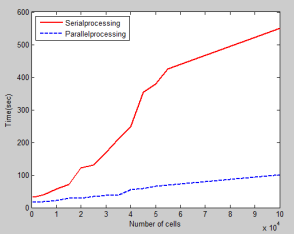Figure 2. Serial vs. parallel processing

The parallel processing code developed has been simulated in various newer version Intel processors which is used to analyze the performance. The number of iterations are kept constant and the cell size is varied and the code is simulated in different Intel processors include i3, i5, i7 and Pentium processors. The results shows that the updated newer version i7 was able to process the data more quickly, so that the parallel processing can be used more efficiently in higher versions system which is easily available now a days. Figure 3 shows the Comparision with different versions of the processors. From the results it is observed that the i7 processor was able to process the code more efficiently than the others. Pentium processor which is one of the oldest among took larger time to process the code.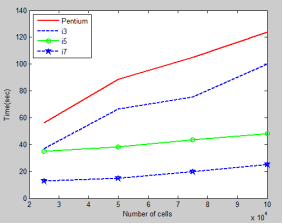Figure 3. Parallel processing on different versions of Intel processors

The parallel code is simulated with different number of Matlab workers. Figure 4 shows the relation between the parallel code and the number of Matlab workers. In this code the number of iterations is kept constant and the time taken by the code to run with different number of cells is noted. The graph shows that parallel code works more efficiently when the number of workers is increased to be four.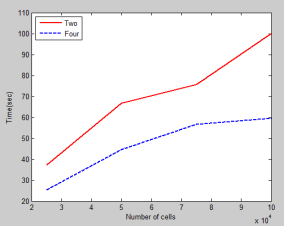Figure 4. Performance with different number of Matlab workers

The speed-ups increase with the problem size because of the better exploitation of CPU resources and parallel processing. Figure 5 shows the achieved speed-ups for with respect to the serial algorithm running on the CPU. Thus the parallel processing gives a better speed up for larger problems.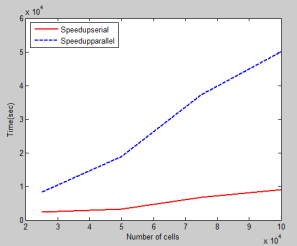Figure 5. Speed up comparison for serial and parallel processing

From the figure 5 it is noted that the parallel processing gives a better speed up. With the previous results the number of iterations and the cell size is varied and the results are noted. For each cell size the iterations are changed and the values are noted. Figure 6 shows the speed up for different cell size at various iterations. From the figure it can be absorbed that larger the computation domain better the speed up thereby making the parallel processing very much suitable for larger computation domain.Figure 6. Iteration vs. Speedup

## CONCLUSION

From the results it is observed that the disadvantage of the FDTD method can be overcome by using Parallel processing FDTD method. The performance analysis of this paper thereby shows that the parallel processing can be easily achieved efficiently by using modern CPU’s present today which can be used to do complex computations.

## REFERENCES

. Yee, K. S., Numerical solution of initial boundary value problems involving Maxwell’s equations in isotropic media,” IEEE Transactions on Antennas and Propagation, Vol. 14, No. 5, 302-307, May 1966.

. Time Domain Maxwell ‘s Equations Solver: Software and User ‘s Guide, Norwood, MA, Artech House, 2004.

. W. Yu and R. Mittra, “A Conformal FDTD Software Package for Modeling of Antennas and Microstrip Circuit Components,” IEEE Antennas and Propagation Magazine, 42, 5, October 2000, pp. 28-39.

. W. Yu and R. Mittra, “A Conformal Finite Difference Time Domain Technique for Modeling Curved Dielectric Surfaces,” IEEE Microwave and Guided Wave Letters, January 2001, pp. 25-27.

. W. Yu and R. Mittra, “A New Sub gridding Method for Finite Difference Time Domain (FDTD) Algorithm,” Microwave Opt. Techno. Lett. , 21, 5, June 1999, pp. 3 3 0-333.

. B. Wang, Y. Wang, W. Yu, and R. Mittra, “A Hybrid 2-D FDTD Sub gridding Scheme for Modeling On-Chip Interconnects,” IEEE Transactions on Advanced Packaging, 24, 4, November 200 1 , pp. 528-533.

. X. Zhu, T. Dogaru, and L. Carin, “Three-Dimensional Biorthogonal Multiresolution Time-Domain Method and Its Application to Electromagnetic Scattering Problems,” IEEE Transactions on Antennas and Propagation, AP-51, 5, May 2003, pp. 1085- 1 092.

. Q. H. Liu, “The PSTD Algorithm: A Time-Domain Method Requiring Only Two Cells Per Wavelength,” Microwave Opt. Technol. Lett.,IS,1997, pp. 1 58- 1 65.

 W. H. Yu, Y. J. Liu, T. Su, N.-T. Huang, and R. Mittra, ”A robust parallel conformal finite-difference time-domain processing package using the MPI library,” IEEE Antennas & Propagat. Mag., vol. 47, no. 3, pp. 39-59, 2005.

 Almasi, G.S, and Gohlied, A, “Highly Parallel Computing”. Benjamin Cummings Publishing, 2a ed., 1994.

. Taflove A, Brodwin ME. Numerical solution of steady-state electromagnetic scattering problems using the time-dependent Maxwell’s equations. IEEE Trans Microwave Theory Tech. 1975,MTT-23(8):623–30.

. W. Yu, X. Yang, Y. Liu, and R. Mittra “Parallel FDTD Performance Analysis on Different Hardware Platforms “,IEEE Int. Symp. Antennas and Propagation Meeting

View all

## DMCA / Removal Request

If you are the original writer of this essay and no longer wish to have your work published on UKEssays.com then please:

Related Services

Prices from

OMR57

Approximate costs for:

• 1000 words
• 7 day delivery

Related Lectures